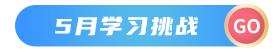1:JAVA语言中，把以下符号称为算术操作符

➕ 加 加法运算
➖ 减 减法运算
※ 乘 乘法运算
/ 除 除法运算（不保留小数）
% 模 模运算（求余数）
2：两个int类型算术运算后，结果仍为int型
3：整形的除法运算（不保留小数部分）
14/5的值为2；
System.out.println("结果为：”+（14/5））；
4：整形的模运算（求余数）
System.out.println("结果为：”+（14%5））；
14%5的值为4

1：浮点型的算术运算

1：当int类型和double类型混合运算时，把int提升为double类型来运算。

（1）概念
＜ 小于
<= 小于等于
＞ 大于
＞= 大于等于
＝＝ 等于
！= 不等于
（2）例子

``````public class java2 {
public static void main(String[] args) {

int a=6,b=4;
boolean result=a>b;
System.out.println("the result: "+result);
}
}
``````

1：

（1）&& 逻辑与 并且

a 为true, b 为true ,必须a,b两者均为true,结果为true

（2）|| 逻辑或 或者

a为true或者b为true，只要满足a,b其中一个为true ，结果为true

(3)！ 逻辑非 非

1：
a=b+100;

（2）：将等号右边表达式的值，赋给左边的变量；

2：区分初始化和赋值

int a=1;//初始化
a=12;//赋值

(1)后置自增：（后自增）
int b=10;
a=b++;

``````public class java2 {
public static void main(String[] args) {

int b=10;
int a;
a=b++;
System.out.println("a的值是："+a+",b的值是："+b);
}
}

``````

(2)前置自增：（先自增）
int b=10;
a=++b;
a=11,b=11

``````public class java2 {
public static void main(String[] args) {

int b=10;
int a;
a=b++;
System.out.println("a的值是："+a+",b的值是："+b);
}
}

``````

（1）积极使用小括号，显式决定谁先谁后

（1）：小数转换成整数的时候，小数部分直接被截断，所以不存在四舍五入。
（2）：默认的其他类型是不支持转换的。例如boolean不能转换成int类型

``````public class java2 {
public static void main(String[] args) {

double a=12.66;
int b;
b=(int) a;
System.out.println("b的值是："+b);
}
}
``````